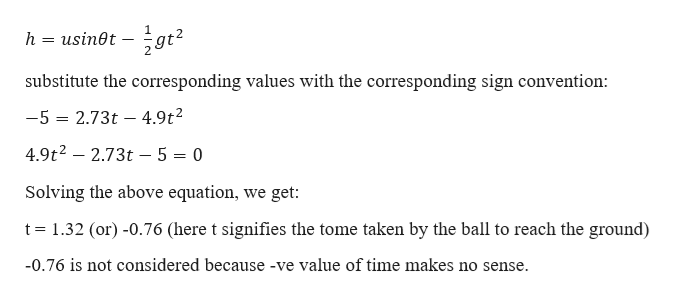# A ball is fired at a speed of 8m/s at 20° above the horizontal from the top of a 5m high wall. How far from the base of the wall will the ball hit the ground?

Question
3 views

A ball is fired at a speed of 8m/s at 20° above the horizontal from the top of a 5m high wall. How far from the base of the wall will the ball hit the ground?

check_circle

star
star
star
star
star
1 Rating
Step 1

Given information:

Initial velocity of the ball (u) = 8 m/s

Vertical component of the velocity (usinθ) = 2.73 m/s

Horizontal component of the velocity (ucosθ) = 7.51 m/s

Angle made by the velocity vector with the horizontal (θ) = 200

The height of the wall (h) = 5 m

Consider the upward direction as “+ve” and downward direction as “-ve”

Step 2

Now consider 2nd equation of motion,...help_outlineImage Transcriptionclosegt usin0t - h substitute the corresponding values with the corresponding sign convention: -5 2.73t 4.9t2 = 4.9t2- 2.73t - 5 = 0 Solving the above equation, we get: t 1.32 (or) -0.76 (here t signifies the tome taken by the ball to reach the ground) -0.76 is not considered because -ve value of time makes no sense. fullscreen

### Want to see the full answer?

See Solution

#### Want to see this answer and more?

Solutions are written by subject experts who are available 24/7. Questions are typically answered within 1 hour.*

See Solution
*Response times may vary by subject and question.
Tagged in

### Kinematics